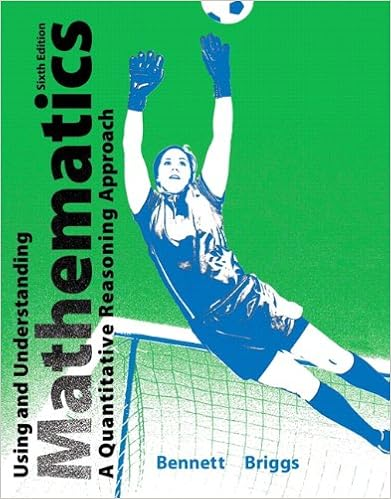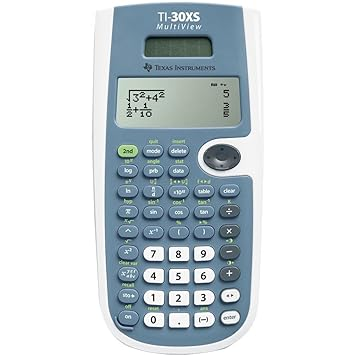Mathematics

Algebra IIISBN# 9780030358296 and TI-84 Calculator

Concepts & Applications (MTH 121B)ISBN-10: 0321914627All students must have a TI – 30XS calculator for this course.

College Algebra/StatisticsISBN# 9780321640314 and TI-84 CalculatorISBN# 9781259345289 and a TI-84 Calculator

Precalculus/TrigonometryISBN# 9780547219929 and TI-84 Calculator

AP Calculus AB and BCISBN# 9780547212906 and TI-84 Calculator

Summer 2018 AP Calculus  AB Work –  2019 Calculus Summer Assignment - 33 downloads